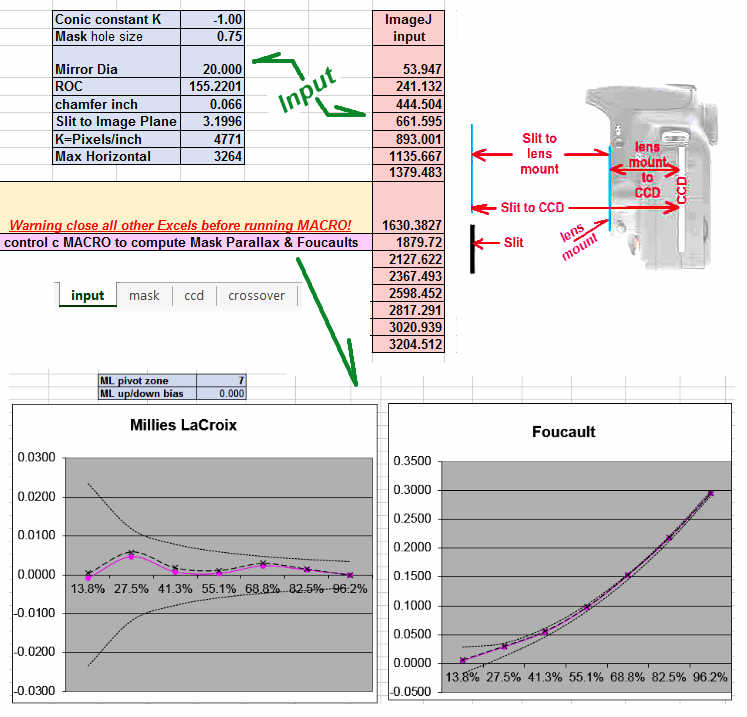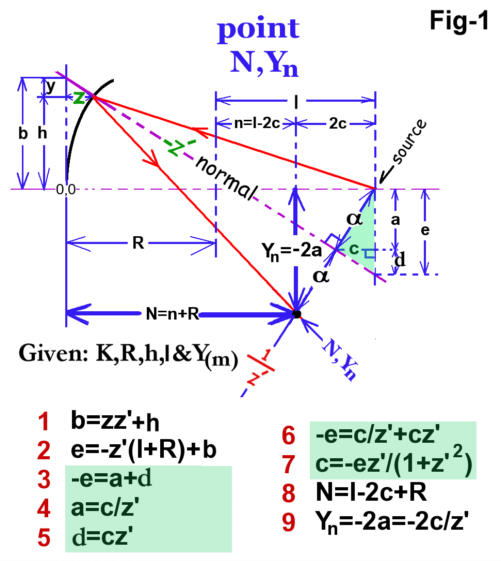Slit Image Test Overview Computations Setup Baldwin On SIT ContactThe spreadsheet can be obtained by send a request to Bill ThomasJeff Baldwin's youtube video - taking a SIT imageJeff Baldwin's youtube video - processing a SIT imageclick to download the 24 mega byte imageJ input tif file to be used with SIT spreadsheet Olympus E300 Evolt Lens mount to CCD: 38.8 mm Pixels/inch: 4771 Canon T2i EOS Rebel Lens mount to CCD: 44 mm Pixels/inch: 5905SIT Nonlinear Algebraic Equations SIT is a Fixed Source Test and as such, the angle of the source ray s is equal to the angle of reflection ray r as measured with respect to the Normal per Fig-1: Alpha represents the angle s and r. The slope of the Normal is -z' per Fig-4. The slope of a perpendicular to the Normal is +1/z' per Fig-3. The Normal vector (per Fig-1 equation 2) of the form y=mx+b is y=-z'x+zz'+h, where zz'+h is the b term. Knowing z, the other side of that right triangle is found using similar triangles where the derivative z' serves as the other triangle with similar sides. Derivative z' and 1/z' are used in many places in Fig-1 in this manner which has greatly simplified this new version of the SIT equations, which gets exactly the same results as the old. Excels Solver (Root finder) is used to find the numerical solution to the SIT nonlinear algebraic equations, which can be simply summarized as: z is varied (using a Root finder) until Yz of Fig-2 equation 12 is nearly equals to Ym (the slit images as measured by ImageJ). Instead of using Z=-Kz of Fig-4 to compute the Foucault, Z=(h-Rz'+zz')/z' is used instead being it avoids multiplying by K=-0 when the conic is a sphere.References
 (1)   Karine and Jean-MarcLecleire, A Manual for Amateur Telescope Making. http://www.willbell.com/tm/atmmanual/index.htm (2)  Conics Optical Shop Testing Edited by Daniel Malacara, Wiley and Sons, 1978, Appendix 1 page 479, 482 Conic approximation Conic equations Conic constant - Schwarzschild constant (3)  LWT J. Francis, "Improved Test Methods for Elliptical and Spherical TCT Mirrors,", ATM J. 14, 34-39, (ISSN 1074-2697). Unfortunately, references to the LWT on the Internet have mostly faded away. Jim Burrows author of Sixtests.exe does mention the LWT in passing at http://www.raddobs.com/atm/atm_math.htm. LWT was a variant of the moving source Caustic where instead of trying to determine a Caustic zone holes focus laterally and longitudinally precisely, the LWT measurements were done manually in the same lateral plane. Being that for the LWT the longitudinal value was irrelevant, didn't require a mask and little judgment was required to determine laterally where a zone ray crossed the lateral plane, the LWT was an improvement over the standard Caustic. However, the required lateral accuracy required was very tight. Given small room temperature and the time to take the measurements, I could not get repeatable LWT results. (4)   Lens mount to CCD spacing    Recommend saving this webpage to your computer, since it has vanished in the past.2005 - 2019 Bill Thomas This work is licensed under a Creative Commons Attribution-NonCommercial-ShareAlike 4.0 International License.